# How to use the T Function in Excel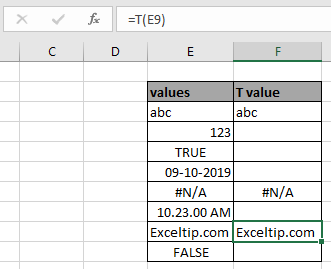In this article, we will learn about how to use the T function in Excel to return only text values or return blank cell otherwise.

What is the T function?

T function in excel returns text value for only the value in text format. A number can be in text format.

Syntax of T function:

=T ( value )

value : value can be given directly or using cell reference

Example:

All of these might be confusing to understand. So, let's test this formula via running it on the example shown below. Here we have some random data values to test the T function in Excel.

Here we have some random values in different cell and we need to get the numerical value in number format using the below formula

Use the formula:

=T(E3)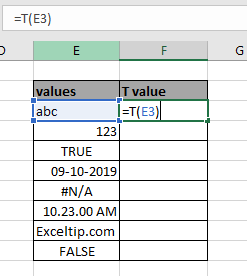Press Enter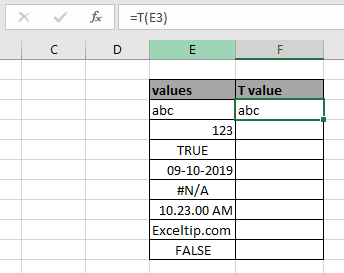As you can see the formula returns text value for the input text value. You can copy the formula from one to the remaining cells using the Ctrl + D shortcut key or using the drag down option in excel.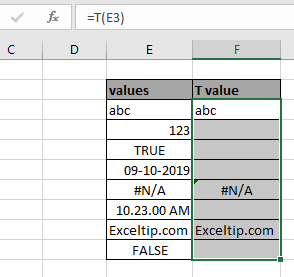As you can see the formula returns blank cell for number, logical value but returns text for text.

Number in text format:

Any value which is in text format, T function returns the number in text format.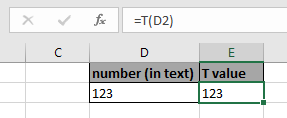Here are some observational notes shown below.

Notes:

1. The formula returns text for the input text value.
2. Any value in text format returns in text format.
3. Returns Caution if no value is input.
4. Blank cell is returned in case of number value as argument.

Hope this article about How to use the T function in Excel is explanatory. Find more articles on the info function here. If you liked our blogs, share it with your fristarts on Facebook. And also you can follow us on Twitter and Facebook. We would love to hear from you, do let us know how we can improve, complement or innovate our work and make it better for you. Write to us at T@exceltip.com.

Related Articles

How to use the CELL function in Excel : returns Information about a cell in a worksheet using the CELL function.

How to use the TYPE Function in Excel : returns numeric code representing the TYPE of data using TYPE function in Excel.

How to use the SHEETS function in Excel : returns a number, reference to input sheet indicated using the SHEETS function.

How to Use ISNUMBER Function in Excel : ISNUMBER function returns TRUE if number or FALSE if not in Excel.

Popular Articles:

50 Excel Shortcuts to Increase Your Productivity | Get faster at your task. These 50 shortcuts will make you work even faster on Excel.

The VLOOKUP Function in Excel | This is one of the most used and popular functions of excel that is used to lookup value from different ranges and sheets.

COUNTIF in Excel 2016 | Count values with conditions using this amazing function. You don't need to filter your data to count specific values. Countif function is essential to prepare your dashboard.

How to Use SUMIF Function in Excel | This is another dashboard essential function. This helps you sum up values on specific conditions.

Terms and Conditions of use

The applications/code on this site are distributed as is and without warranties or liability. In no event shall the owner of the copyrights, or the authors of the applications/code be liable for any loss of profit, any problems or any damage resulting from the use or evaluation of the applications/code.